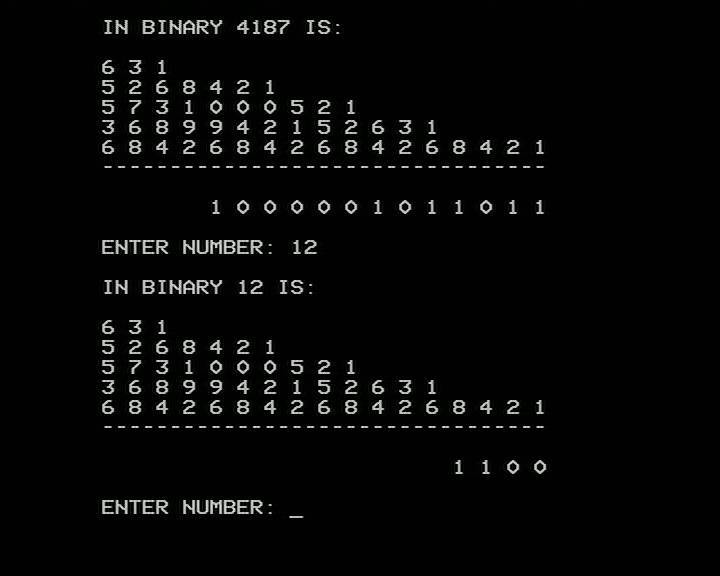Working with BinaryThinking of each digit in a binary number as a column, going from right to left the column holds a decimal value of 1 and then doubles for each column. A binary 0 indicates that the column is clear, 1 means it is set. So to convert a binary number to decimal, we can lay out column headings like shown above and add up the decimal value for each column where the binary digit is 1. So 1100 in binary is 8+4+0+0=12 decimal.